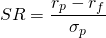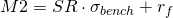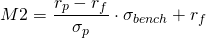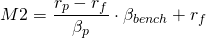# M2 measure

The m2 measure, also known as the Modigliani risk-adjusted performance measure, is a risk-adjusted performance measure. It is closely related to the Sharpe ratio, but does not have the downside of being ‘dimensionless’ measure. Moreover, in case of negative returns, the m2 measure continues to hold its meaning, while the Sharpe ratio very hard to interpret.

## M2 measure calculation

The main question we want to address first is; how to measure m2? Practically, calculating the m2 measure is not more difficult than measuring the Sharpe ratio.

## step 1

First of all, we need to calculate the Sharpe ratio. In a next step, we will use the Sharpe ratio to derive the m2 measure. Thus, first we perform the following calculation, which is just the standard (annual) Sharpe ratio equation.## step 2

The second step consists of multiplying the Sharpe ratio by the (annualized) standard deviation of a benchmark. The benchmark can be freely chosen, e.g. the S&P500 index, the MSCI World index, or another broad index.

## step 3

In a last step, we add the risk free rate is againWe can rewrite the above measure differently to get a better understanding of what the m2 measure captures. As shown in the equation below, the M2 measure equals the excess return weighted over the benchmark’s and portfolio’s standard deviation and increased with the risk free return. Clearly, the M2 measure just equals the portfolio’s return when had the standard deviation of the portfolio equaled that of the benchmark. This is the case when happens when the portfolio is simply tracking an index## Alternative M2 measure

There is also an alternative m2 measure that is sometimes computed by practioners. In this case, the systematic risk component is used instead of the full volatility component. However, this is only be a good indicator when the portfolio is well diversified. Underdiversification may result in an underestimation of the riskiness of the portfolio, as their may be some idiosyncratic risk left!## Summary

The m2 measure is an alternative and easy to interpret risk-adjusted performance metric. It is closely related to the Sharpe ratio, from which it is derived.